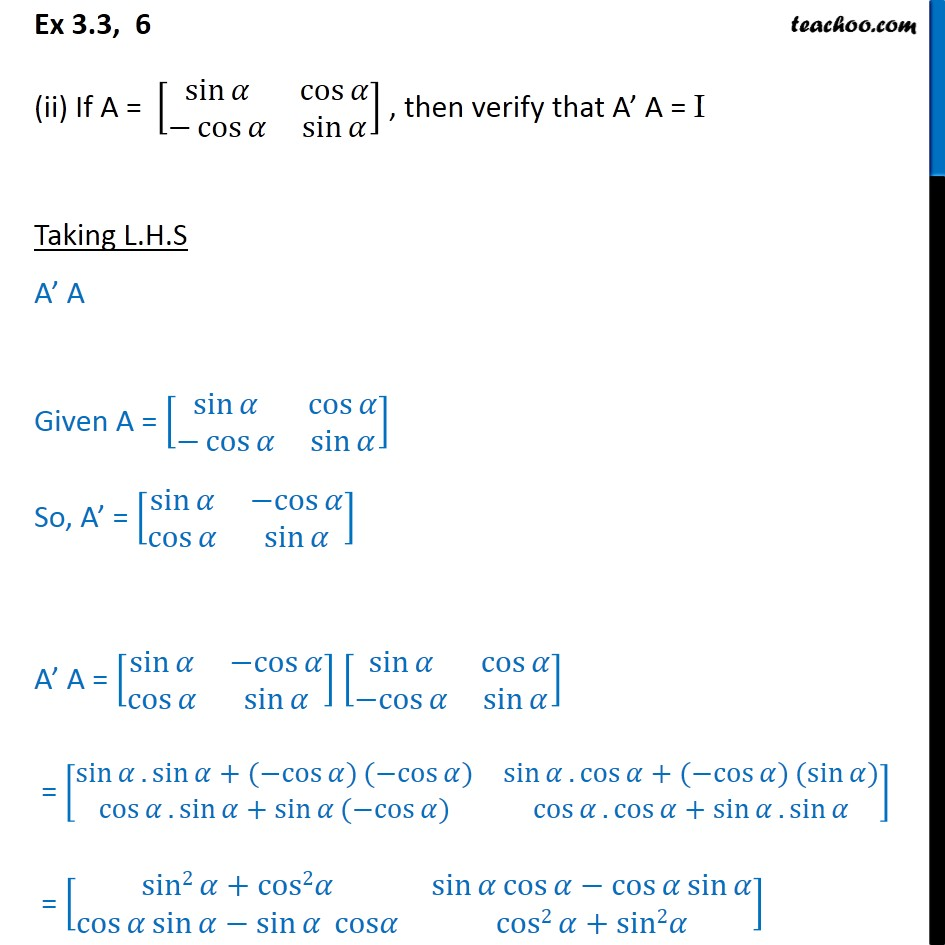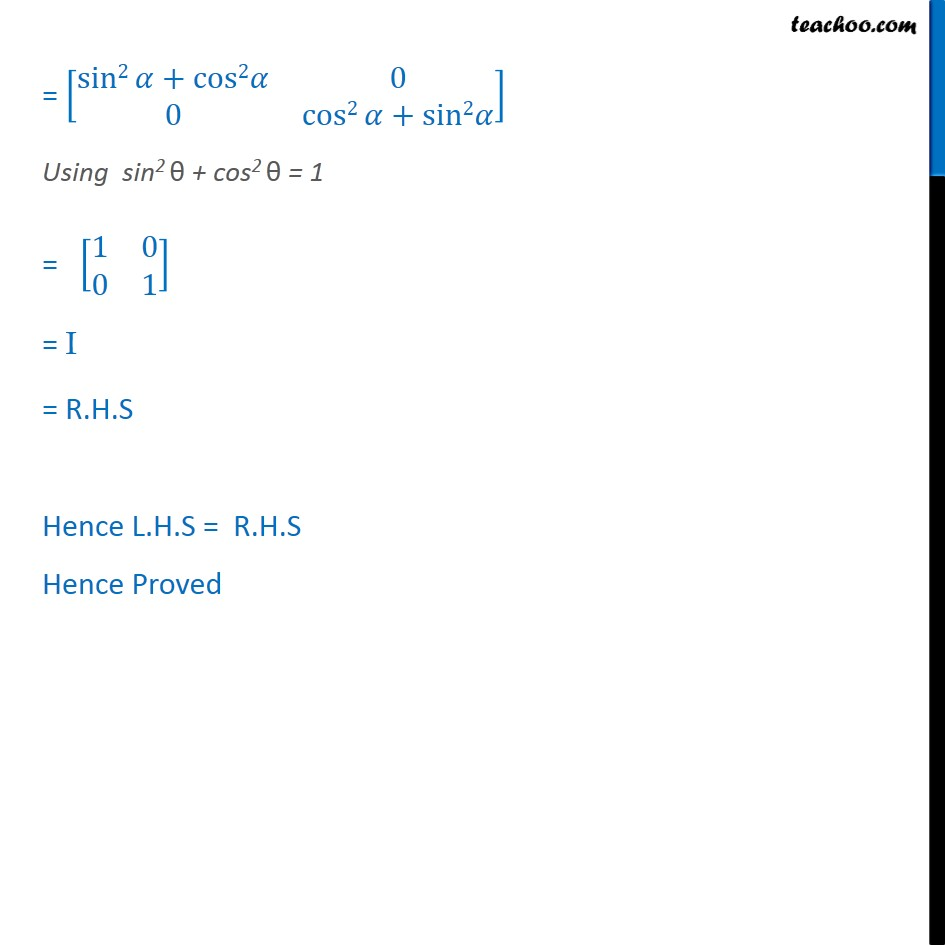Ex 3.3

Chapter 3 Class 12 Matrices
Serial order wiseGet live Maths 1-on-1 Classs - Class 6 to 12

### Transcript

Ex 3.3, 6 (ii) If A = [ 8(sin &cos @ cos &sin )] , then verify that A A = I Taking L.H.S A A Given A = [ 8(sin &cos @ cos &sin )] So, A = [ 8(sin & cos @cos &sin )] A A = [ 8(sin & cos @cos &sin )] [ 8(sin &cos @ cos &sin )] = [ 8(sin .sin + ( cos ) ( cos ) &sin .cos + ( cos ) (sin ) @cos .sin +sin ( cos ) &cos .cos +sin .sin )] = [ 8(sin2 +cos2 &sin cos cos sin @cos sin sin cos &cos2 +sin2 )] = [ 8(sin2 +cos2 &[email protected]&cos2 +sin2 )] Using sin2 + cos2 = 1 = [ 8(1&[email protected]&1)] = I = R.H.S Hence L.H.S = R.H.S Hence Proved